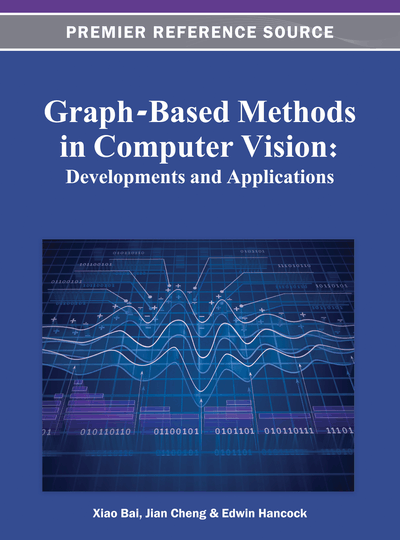# Geometric-Edge Random Graph Model for Image Representation

Jiang Bo (Anhui University, China), Tang Jing (Anhui University, China) and Luo Bin (Anhui University, China)
DOI: 10.4018/978-1-4666-1891-6.ch002
Available
\$37.50
No Current Special Offers

## Abstract

This chapter presents a random graph model for image representation. The first contribution the authors propose is a Geometric-Edge (G-E) Random Graph Model for image representation. The second contribution is that of casting image matching into G-E Random Graph matching by using the random dot product graph based matching algorithm. Experimental results show that the proposed G-E Random Graph model and matching algorithm are effective and robust to structural variations.
Chapter Preview
Top

## Geometric-Edge (Ge) Random Graph Model

In this section, we develop the G-E Random Graph model starting from the general geometric graph. As a traditional graph model, geometric graph can be used to extract structure information of an image.

## Complete Chapter List

Search this Book:
Reset